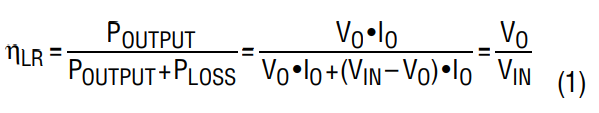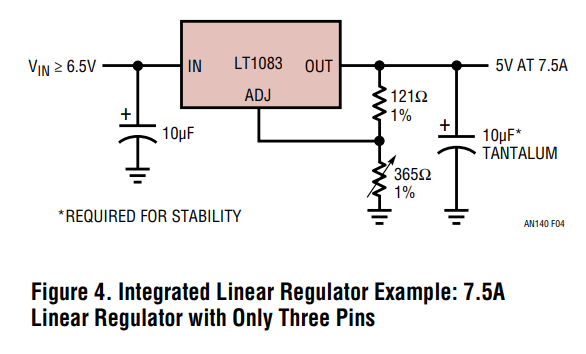# Linear Regulator Calculator

## This tool calculates properties of a linear regulator.

Inputs
Output Voltage
Resistor R1
Resistor R2
Reference Voltage
Tolerance
Joules

Output
Minimum Output Voltage
Maximum Output Voltage

### Equations

Using linear regulators can be the excessive power dissipation of its series transistor Q1 operating in a linear model. The linear regulator transistor is conceptually a variable resistor.

Since all the load current must pass through the series transistor, its power dissipation is $$PLoss = (VIN – VO) • IO$$. In this case, the efficiency of a linear regulator can be quickly estimated by:### Applications

The linear regulator has been widely used by industry until now in a wide range of applications. Power management suppliers have developed many integrated linear regulators.
A typical integrated linear regulator needs only VIN, VOUT, FB and optional GND pins. Figure below shows a typical 3-pin linear regulator, the LT1083, which was developed more than 20 years ago by Analog Devices. It only needs an input capacitor, output capacitor and two feedback resistors to set the output voltage. Almost any electrical engineer can design a supply with these simple linear regulators.Integrated Linear Regulator Example: 7.5A Linear Regulator with Only Three Pins